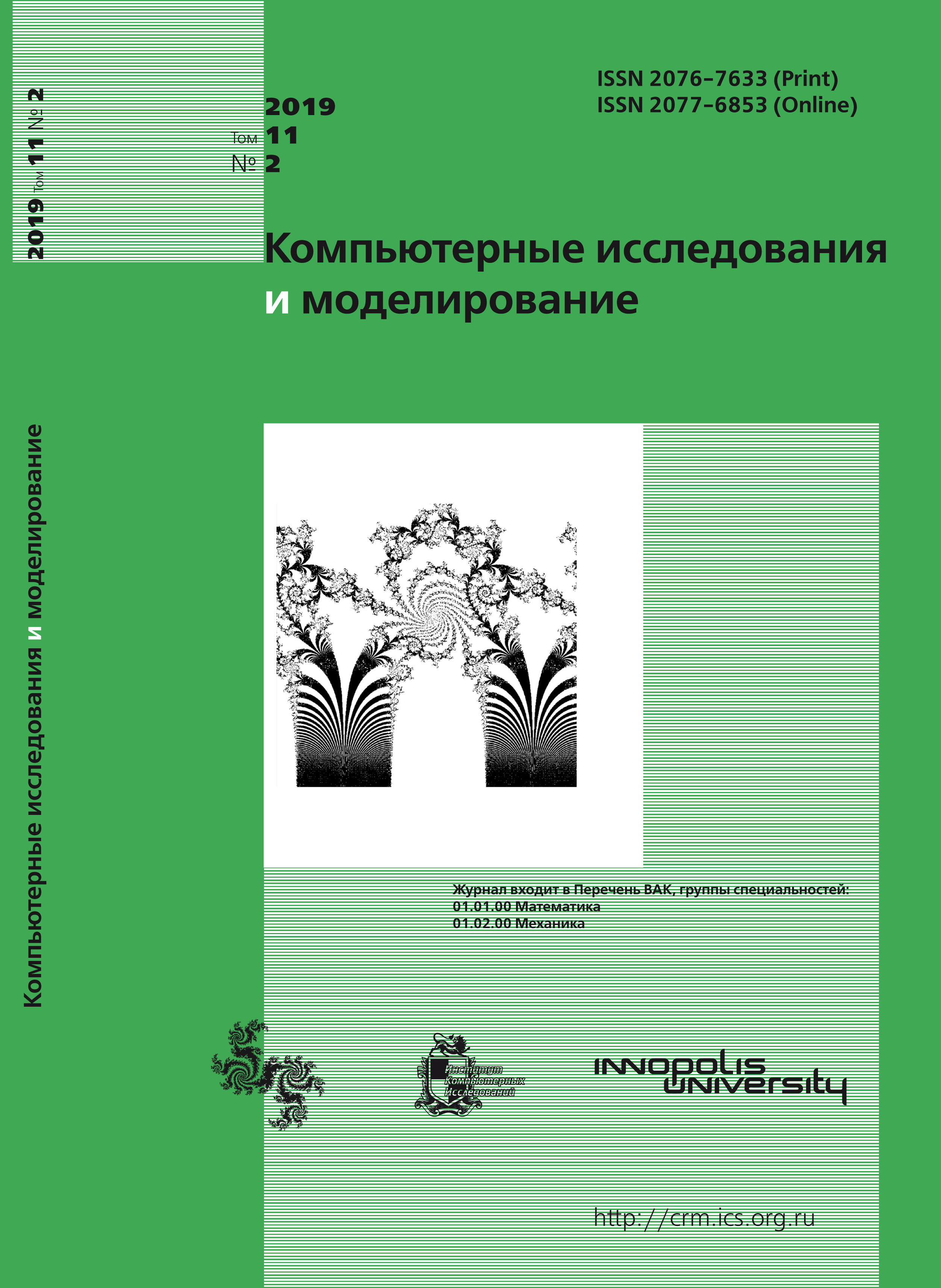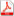Issue 2, 2019 Vol. 11

# All issues

On some stochastic mirror descent methods for constrained online optimization problemspdf (153K)  / List of references

The problem of online convex optimization naturally occurs in cases when there is an update of statistical information. The mirror descent method is well known for non-smooth optimization problems. Mirror descent is an extension of the subgradient method for solving non-smooth convex optimization problems in the case of a non-Euclidean distance. This paper is devoted to a stochastic variant of recently proposed Mirror Descent methods for convex online optimization problems with convex Lipschitz (generally, non-smooth) functional constraints. This means that we can still use the value of the functional constraint, but instead of (sub)gradient of the objective functional and the functional constraint, we use their stochastic (sub)gradients. More precisely, assume that on a closed subset of $n$-dimensional vector space, $N$ convex Lipschitz non-smooth functionals are given. The problem is to minimize the arithmetic mean of these functionals with a convex Lipschitz constraint. Two methods are proposed, for solving this problem, using stochastic (sub)gradients: adaptive method (does not require knowledge of Lipschitz constant neither for the objective functional, nor for the functional of constraint) and non-adaptivemethod (requires knowledge of Lipschitz constant for the objective functional and the functional of constraint). Note that it is allowed to calculate the stochastic (sub)gradient of each functional only once. In the case of non-negative regret, we find that the number of non-productive steps is $O$($N$), which indicates the optimality of the proposed methods. We consider an arbitrary proximal structure, which is essential for decisionmaking problems. The results of numerical experiments are presented, allowing to compare the work of adaptive and non-adaptive methods for some examples. It is shown that the adaptive method can significantly improve the number of the found solutions.

Keywords: online convex optimization problem, non-smooth constrained optimization problem, adaptive mirror Descent, Lipschitz functional, Stochastic (sub)gradient
Citation in English: Alkousa M.S. On some stochastic mirror descent methods for constrained online optimization problems // Computer Research and Modeling, 2019, vol. 11, no. 2, pp. 205-217
Citation in English: Alkousa M.S. On some stochastic mirror descent methods for constrained online optimization problems // Computer Research and Modeling, 2019, vol. 11, no. 2, pp. 205-217
DOI: 10.20537/2076-7633-2019-11-2-205-217
Views (last year): 42.

Full-text version of the journal is also available on the web site of the scientific electronic library eLIBRARY.RU

The journal is included in the Russian Science Citation Index

The journal is included in the List of Russian peer-reviewed journals publishing the main research results of PhD and doctoral dissertations.

International Interdisciplinary Conference "Mathematics. Computing. Education"

The journal is included in the RSCI

Indexed in Scopus